Anzeige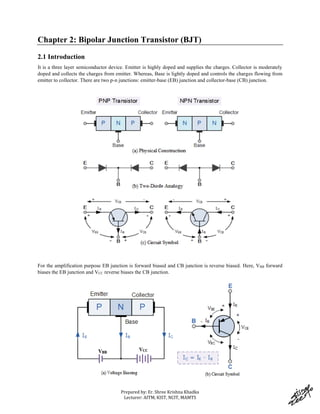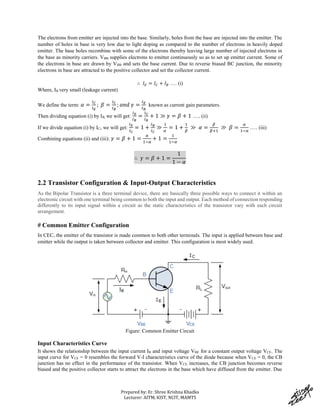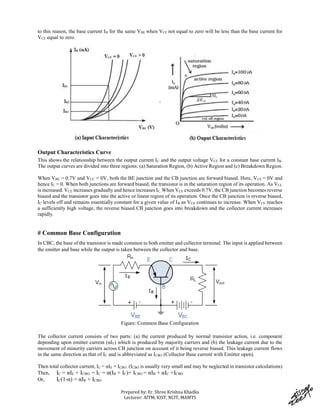Anzeige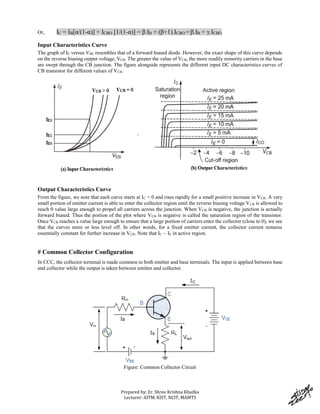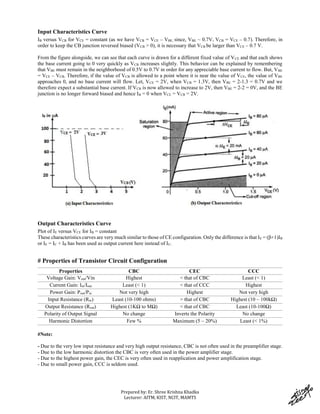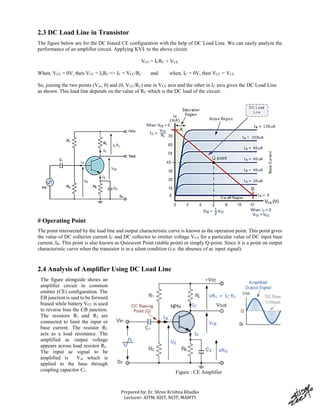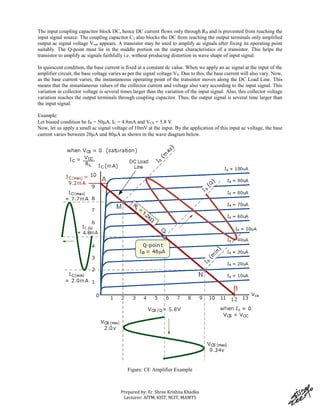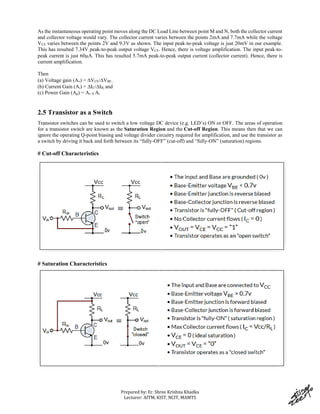Anzeige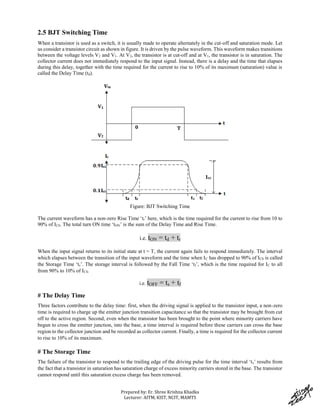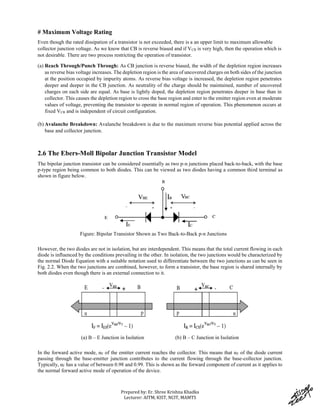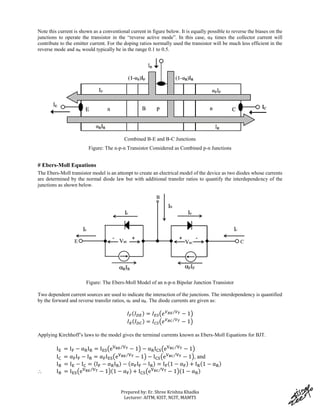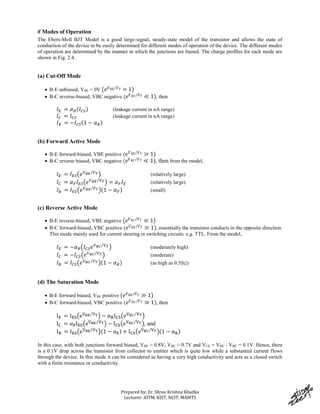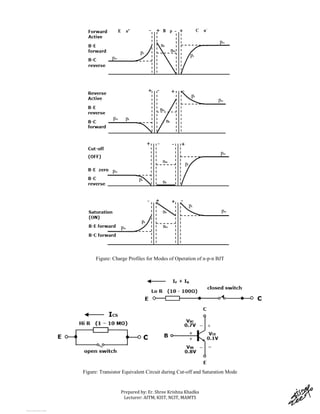Nächste SlideShare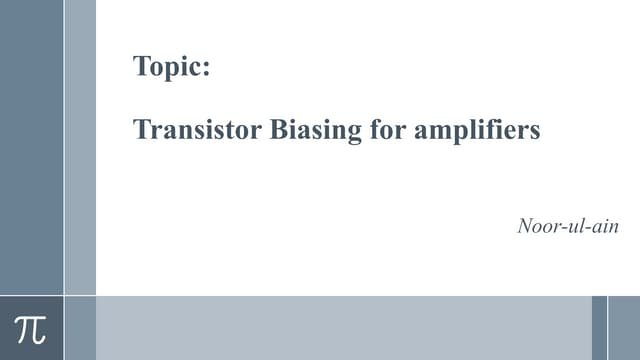Electronics and modern physics presentation
1 von 14
Anzeige

### Chapter2BipolarJunctionTransistor (1).pdf

1. See discussions, stats, and author profiles for this publication at: https://www.researchgate.net/publication/323384291 Bipolar Junction Transistor Chapter · February 2018 CITATIONS 0 READS 34,876 1 author: Some of the authors of this publication are also working on these related projects: Information & Communication Engineering Courses View project Research Proposal and Proceedings View project Shree Krishna Khadka Nepal Telecom 45 PUBLICATIONS 151 CITATIONS SEE PROFILE All content following this page was uploaded by Shree Krishna Khadka on 24 February 2018. The user has requested enhancement of the downloaded file.
2. Prepared by: Er. Shree Krishna Khadka Lecturer: AITM, KIST, NCIT, MAMTS Chapter 2: Bipolar Junction Transistor (BJT) 2.1 Introduction It is a three layer semiconductor device. Emitter is highly doped and supplies the charges. Collector is moderately doped and collects the charges from emitter. Whereas, Base is lightly doped and controls the charges flowing from emitter to collector. There are two p-n junctions: emitter-base (EB) junction and collector-base (CB) junction. For the amplification purpose EB junction is forward biased and CB junction is reverse biased. Here, VBB forward biases the EB junction and VCC reverse biases the CB junction.
3. Prepared by: Er. Shree Krishna Khadka Lecturer: AITM, KIST, NCIT, MAMTS The electrons from emitter are injected into the base. Similarly, holes from the base are injected into the emitter. The number of holes in base is very low due to light doping as compared to the number of electrons in heavily doped emitter. The base holes recombine with some of the electrons thereby leaving large number of injected electrons in the base as minority carriers. VBB supplies electrons to emitter continuously so as to set up emitter current. Some of the electrons in base are drawn by VBB and sets the base current. Due to reverse biased BC junction, the minority electrons in base are attracted to the positive collector and set the collector current. ∴ 𝐼𝐸 = 𝐼𝐶 + 𝐼𝐵 …. (i) Where, IB very small (leakage current) We define the term: 𝛼 = 𝐼𝐶 𝐼𝐸 ; 𝛽 = 𝐼𝐶 𝐼𝐵 ; 𝑎𝑛𝑑 𝛾 = 𝐼𝐸 𝐼𝐵 known as current gain parameters. Then dividing equation (i) by IB, we will get: 𝐼𝐸 𝐼𝐵 = 𝐼𝐶 𝐼𝐵 + 1 ≫ 𝛾 = 𝛽 + 1 …. (ii) If we divide equation (i) by IC, we will get: 𝐼𝐸 𝐼𝐶 = 1 + 𝐼𝐵 𝐼𝐶 ≫ 1 𝛼 = 1 + 1 𝛽 ≫ 𝛼 = 𝛽 𝛽+1 ≫ 𝛽 = 𝛼 1−𝛼 …. (iii) Combining equations (ii) and (iii): 𝛾 = 𝛽 + 1 = 𝛼 1−𝛼 + 1 = 1 1−𝛼 ∴ 𝛾 = 𝛽 + 1 = 1 1 − 𝛼 2.2 Transistor Configuration & Input-Output Characteristics As the Bipolar Transistor is a three terminal device, there are basically three possible ways to connect it within an electronic circuit with one terminal being common to both the input and output. Each method of connection responding differently to its input signal within a circuit as the static characteristics of the transistor vary with each circuit arrangement. # Common Emitter Configuration In CEC, the emitter of the transistor is made common to both other terminals. The input is applied between base and emitter while the output is taken between collector and emitter. This configuration is most widely used. Figure: Common Emitter Circuit Input Characteristics Curve It shows the relationship between the input current IB and input voltage VBE for a constant output voltage VCE. The input curve for VCE = 0 resembles the forward V-I characteristics curve of the diode because when VCE = 0, the CB junction has no effect in the performance of the transistor. When VCE increases, the CB junction becomes reverse biased and the positive collector starts to attract the electrons in the base which have diffused from the emitter. Due
4. Prepared by: Er. Shree Krishna Khadka Lecturer: AITM, KIST, NCIT, MAMTS to this reason, the base current IB for the same VBE when VCE not equal to zero will be less than the base current for VCE equal to zero. Output Characteristics Curve This shows the relationship between the output current IC and the output voltage VCE for a constant base current IB. The output curves are divided into three regions: (a) Saturation Region, (b) Active Region and (c) Breakdown Region. When VBE = 0.7V and VCC = 0V, both the BE junction and the CB junction are forward biased. Here, VCE = 0V and hence IC = 0. When both junctions are forward biased, the transistor is in the saturation region of its operation. As VCC is increased. VCE increases gradually and hence increases IC. When VCE exceeds 0.7V, the CB junction becomes reverse biased and the transistor goes into the active or linear region of its operation. Once the CB junction is reverse biased, IC levels off and remains essentially constant for a given value of IB as VCE continues to increase. When VCE reaches a sufficiently high voltage, the reverse biased CB junction goes into breakdown and the collector current increases rapidly. # Common Base Configuration In CBC, the base of the transistor is made common to both emitter and collector terminal. The input is applied between the emitter and base while the output is taken between the collector and base. Figure: Common Base Configuration The collector current consists of two parts: (a) the current produced by normal transistor action, i.e. component depending upon emitter current (αIE) which is produced by majority carriers and (b) the leakage current due to the movement of minority carriers across CB junction on account of it being reverse biased. This leakage current flows in the same direction as that of IC and is abbreviated as ICBO (Collector Base current with Emitter open). Then total collector current, IC = αIE + ICBO. (ICBO is usually very small and may be neglected in transistor calculations) Then, IC = αIE + ICBO. = IC = α(IB + IC)+ ICBO = αIB + αIC +ICBO Or, IC(1-α) = αIB + ICBO
5. Prepared by: Er. Shree Krishna Khadka Lecturer: AITM, KIST, NCIT, MAMTS Or, IC = IB[α/(1-α)] + ICBO [1/(1-α)] = β.IB + (β+1).ICBO = β.IB + γ.ICBO Input Characteristics Curve The graph of IE versus VBE resembles that of a forward biased diode. However, the exact shape of this curve depends on the reverse biasing output voltage, VCB. The greater the value of VCB, the more readily minority carriers in the base are swept through the CB junction. The figure alongside represents the different input DC characteristics curves of CB transistor for different values of VCB. Output Characteristics Curve From the figure, we note that each curve starts at IC = 0 and rises rapidly for a small positive increase in VCB. A very small portion of emitter current is able to enter the collector region until the reverse biasing voltage VCB is allowed to reach 0 value large enough to propel all carriers across the junction. When VCB is negative, the junction is actually forward biased. Thus the portion of the plot where VCB is negative is called the saturation region of the transistor. Once VCB reaches a value large enough to ensure that a large portion of carriers enter the collector (close to 0), we see that the curves more or less level off. In other words, for a fixed emitter current, the collector current remains essentially constant for further increase in VCB. Note that IC ~ IE in active region. # Common Collector Configuration In CCC, the collector terminal is made common to both emitter and base terminals. The input is applied between base and collector while the output is taken between emitter and collector. Figure: Common Collector Circuit
6. Prepared by: Er. Shree Krishna Khadka Lecturer: AITM, KIST, NCIT, MAMTS Input Characteristics Curve IB versus VCB for VCE = constant (as we have VCB = VCE – VBE, since, VBE ~ 0.7V, VCB = VCE – 0.7). Therefore, in order to keep the CB junction reversed biased (VCB > 0), it is necessary that VCB be larger than VCE – 0.7 V. From the figure alongside, we can see that each curve is drawn for a different fixed value of VCE and that each shows the base current going to 0 very quickly as VCB increases slightly. This behavior can be explained by remembering that VBE must remain in the neighborhood of 0.5V to 0.7V in order for any appreciable base current to flow. But, VBE = VCE – VCB. Therefore, if the value of VCB is allowed to a point where it is near the value of VCE, the value of VBE approaches 0, and no base current will flow. Let, VCE = 2V, when VCB = 1.3V, then VBE = 2-1.3 = 0.7V and we therefore expect a substantial base current. If VCB is now allowed to increase to 2V, then VBE = 2-2 = 0V, and the BE junction is no longer forward biased and hence IB = 0 when VCE = VCB = 2V. Output Characteristics Curve Plot of IE versus VCE for IB = constant These characteristics curves are very much similar to those of CE configuration. Only the difference is that IE = (β+1)IB or IE = IC + IB has been used as output current here instead of IC. # Properties of Transistor Circuit Configuration Properties CBC CEC CCC Voltage Gain: Vout/Vin Highest < that of CBC Least (< 1) Current Gain: Iin/Iout Least (< 1) < that of CCC Highest Power Gain: Pout/Pin Not very high Highest Not very high Input Resistance (Rin) Least (10-100 ohms) > that of CBC Highest (10 – 100kΩ) Output Resistance (Rout) Highest (1KΩ to MΩ) < that of CBC Least (10-100Ω) Polarity of Output Signal No change Inverts the Polarity No change Harmonic Distortion Few % Maximum (5 – 20%) Least (< 1%) #Note: - Due to the very low input resistance and very high output resistance, CBC is not often used in the preamplifier stage. - Due to the low harmonic distortion the CBC is very often used in the power amplifier stage. - Due to the highest power gain, the CEC is very often used in reapplication and power amplification stage. - Due to small power gain, CCC is seldom used.
7. Prepared by: Er. Shree Krishna Khadka Lecturer: AITM, KIST, NCIT, MAMTS 2.3 DC Load Line in Transistor The figure below are for the DC biased CE configuration with the help of DC Load Line. We can easily analyze the performance of an amplifier circuit. Applying KVL to the above circuit VCC = ICRC + VCE. When, VCE = 0V, then VCC = IcRC => IC = VCC/RC and when, IC = 0V, then VCC = VCE So, joining the two points (Vcc, 0) and (0, VCC/RC) one in VCE axis and the other in IC axis gives the DC Load Line as shown. This load line depends on the value of RC which is the DC load of the circuit. # Operating Point The point intersected by the load line and output characteristic curve is known as the operation point. This point gives the value of DC collector current IC and DC collector to emitter voltage VCE for a particular value of DC input base current, IB. This point is also known as Quiescent Point (stable point) or simply Q-point. Since it is a point on output characteristic curve when the transistor is in a silent condition (i.e. the absence of ac input signal). 2.4 Analysis of Amplifier Using DC Load Line The figure alongside shows an amplifier circuit in common emitter (CE) configuration. The EB junction is said to be forward biased while battery VCC is used to reverse bias the CB junction. The resistors R1 and R2 are connected to limit the input or base current. The resistor RL acts as a load resistance. The amplified ac output voltage appears across load resistor RL. The input ac signal to be amplified is Vin which is applied to the base through coupling capacitor C1. Figure : CE Amplifier
8. Prepared by: Er. Shree Krishna Khadka Lecturer: AITM, KIST, NCIT, MAMTS The input coupling capacitor block DC, hence DC current flows only through RB and is prevented from reaching the input signal source. The coupling capacitor C2 also blocks the DC from reaching the output terminals only amplified output ac signal voltage Vout appears. A transistor may be used to amplify ac signals after fixing its operating point suitably. The Q-point must lie in the middle portion on the output characteristics of a transistor. This helps the transistor to amplify ac signals faithfully i.e. without producing distortion in wave shape of input signal. In quiescent condition, the base current is fixed at a constant dc value. When we apply an ac signal at the input of the amplifier circuit, the base voltage varies as per the signal voltage VS. Due to this, the base current will also vary. Now, as the base current varies, the instantaneous operating point of the transistor moves along the DC Load Line. This means that the instantaneous values of the collector current and voltage also vary according to the input signal. This variation in collector voltage is several times larger than the variation of the input signal. Also, this collector voltage variation reaches the output terminals through coupling capacitor. Thus, the output signal is several time larger than the input signal. Example: Let biased condition be IB = 50A, IC = 4.8mA and VCE = 5.8 V. Now, let us apply a small ac signal voltage of 10mV at the input. By the application of this input ac voltage, the base current varies between 20A and 80A as shown in the wave diagram below. Figure: CE Amplifier Example
9. Prepared by: Er. Shree Krishna Khadka Lecturer: AITM, KIST, NCIT, MAMTS As the instantaneous operating point moves along the DC Load Line between point M and N, both the collector current and collector voltage would vary. The collector current varies between the points 2mA and 7.7mA while the voltage VCE varies between the points 2V and 9.3V as shown. The input peak-to-peak voltage is just 20mV in our example. This has resulted 7.34V peak-to-peak output voltage VCE. Hence, there is voltage amplification. The input peak-to- peak current is just 60A. This has resulted 5.7mA peak-to-peak output current (collector current). Hence, there is current amplification. Then (a) Voltage gain (Av) = VCE/VBE , (b) Current Gain (Ai) = IC/IB, and (c) Power Gain (Ap) = Av X Ai. 2.5 Transistor as a Switch Transistor switches can be used to switch a low voltage DC device (e.g. LED’s) ON or OFF. The areas of operation for a transistor switch are known as the Saturation Region and the Cut-off Region. This means then that we can ignore the operating Q-point biasing and voltage divider circuitry required for amplification, and use the transistor as a switch by driving it back and forth between its “fully-OFF” (cut-off) and “fully-ON” (saturation) regions. # Cut-off Characteristics # Saturation Characteristics
10. Prepared by: Er. Shree Krishna Khadka Lecturer: AITM, KIST, NCIT, MAMTS 2.5 BJT Switching Time When a transistor is used as a switch, it is usually made to operate alternately in the cut-off and saturation mode. Let us consider a transistor circuit as shown in figure. It is driven by the pulse waveform. This waveform makes transitions between the voltage levels V2 and V1. At V2, the transistor is at cut-off and at V1, the transistor is in saturation. The collector current does not immediately respond to the input signal. Instead, there is a delay and the time that elapses during this delay, together with the time required for the current to rise to 10% of its maximum (saturation) value is called the Delay Time (td). Figure: BJT Switching Time The current waveform has a non-zero Rise Time ‘tr’ here, which is the time required for the current to rise from 10 to 90% of ICS. The total turn ON time ‘tON’ is the sum of the Delay Time and Rise Time. i.e. tON = td + tr When the input signal returns to its initial state at t = T, the current again fails to respond immediately. The interval which elapses between the transition of the input waveform and the time when IC has dropped to 90% of ICS is called the Storage Time ‘ts’. The storage interval is followed by the Fall Time ‘tf’, which is the time required for IC to all from 90% to 10% of ICS. i.e. tOFF = ts + tf # The Delay Time Three factors contribute to the delay time: first, when the driving signal is applied to the transistor input, a non-zero time is required to charge up the emitter junction transition capacitance so that the transistor may be brought from cut off to the active region. Second, even when the transistor has been brought to the point where minority carriers have begun to cross the emitter junction, into the base, a time interval is required before these carriers can cross the base region to the collector junction and be recorded as collector current. Finally, a time is required for the collector current to rise to 10% of its maximum. # The Storage Time The failure of the transistor to respond to the trailing edge of the driving pulse for the time interval ‘ts’ results from the fact that a transistor in saturation has saturation charge of excess minority carriers stored in the base. The transistor cannot respond until this saturation excess charge has been removed.
11. Prepared by: Er. Shree Krishna Khadka Lecturer: AITM, KIST, NCIT, MAMTS # Maximum Voltage Rating Even though the rated dissipation of a transistor is not exceeded, there is a an upper limit to maximum allowable collector junction voltage. As we know that CB is reverse biased and if VCB is very high, then the operation which is not desirable. There are two process restricting the operation of transistor. (a) Reach Through/Punch Through: As CB junction is reverse biased, the width of the depletion region increases as reverse bias voltage increases. The depletion region is the area of uncovered charges on both sides of the junction at the position occupied by impurity atoms. As reverse bias voltage is increased, the depletion region penetrates deeper and deeper in the CB junction. As neutrality of the charge should be maintained, number of uncovered charges on each side are equal. As base is lightly doped, the depletion region penetrates deeper in base than in collector. This causes the depletion region to cross the base region and enter to the emitter region even at moderate values of voltage, preventing the transistor to operate in normal region of operation. This phenomenon occurs at fixed VCB and is independent of circuit configuration. (b) Avalanche Breakdown: Avalanche breakdown is due to the maximum reverse bias potential applied across the base and collector junction. 2.6 The Ebers-Moll Bipolar Junction Transistor Model The bipolar junction transistor can be considered essentially as two p-n junctions placed back-to-back, with the base p-type region being common to both diodes. This can be viewed as two diodes having a common third terminal as shown in figure below. Figure: Bipolar Transistor Shown as Two Back-to-Back p-n Junctions However, the two diodes are not in isolation, but are interdependent. This means that the total current flowing in each diode is influenced by the conditions prevailing in the other. In isolation, the two junctions would be characterized by the normal Diode Equation with a suitable notation used to differentiate between the two junctions as can be seen in Fig. 2.2. When the two junctions are combined, however, to form a transistor, the base region is shared internally by both diodes even though there is an external connection to it. (a) B – E Junction in Isolation (b) B – C Junction in Isolation In the forward active mode, αF of the emitter current reaches the collector. This means that αF of the diode current passing through the base-emitter junction contributes to the current flowing through the base-collector junction. Typically, αF has a value of between 0.98 and 0.99. This is shown as the forward component of current as it applies to the normal forward active mode of operation of the device.
12. Prepared by: Er. Shree Krishna Khadka Lecturer: AITM, KIST, NCIT, MAMTS Note this current is shown as a conventional current in figure below. It is equally possible to reverse the biases on the junctions to operate the transistor in the “reverse active mode”. In this case, αR times the collector current will contribute to the emitter current. For the doping ratios normally used the transistor will be much less efficient in the reverse mode and αR would typically be in the range 0.1 to 0.5. Combined B-E and B-C Junctions Figure: The n-p-n Transistor Considered as Combined p-n Junctions # Ebers-Moll Equations The Ebers-Moll transistor model is an attempt to create an electrical model of the device as two diodes whose currents are determined by the normal diode law but with additional transfer ratios to quantify the interdependency of the junctions as shown below. Figure: The Ebers-Moll Model of an n-p-n Bipolar Junction Transistor Two dependent current sources are used to indicate the interaction of the junctions. The interdependency is quantified by the forward and reverse transfer ratios, αF and αR. The diode currents are given as: 𝐼𝐹(𝐼𝐷𝐸) = 𝐼𝐸𝑆(𝑒𝑉𝐵𝐸 𝑉𝑇 ⁄ − 1) 𝐼𝑅(𝐼𝐷𝐶) = 𝐼𝐶𝑆(𝑒𝑉𝐵𝐶 𝑉𝑇 ⁄ − 1) Applying Kirchhoff’s laws to the model gives the terminal currents known as Ebers-Moll Equations for BJT. IE = IF − αRIR = IES(eVBE VT ⁄ − 1) − αRICS(eVBC VT ⁄ − 1) IC = αFIF − IR = αFIES(eVBE VT ⁄ − 1) − ICS(eVBC VT ⁄ − 1), and IB = IE − IC = (IF − αRIR) − (αFIF − IR) = IF(1 − αF) + IR(1 − αR) ⸫ IB = IES(eVBE VT ⁄ − 1)(1 − αF) + ICS(eVBC VT ⁄ − 1)(1 − αR)
13. Prepared by: Er. Shree Krishna Khadka Lecturer: AITM, KIST, NCIT, MAMTS # Modes of Operation The Ebers-Moll BJT Model is a good large-signal, steady-state model of the transistor and allows the state of conduction of the device to be easily determined for different modes of operation of the device. The different modes of operation are determined by the manner in which the junctions are biased. The charge profiles for each mode are shown in Fig. 2.4. (a) Cut-Off Mode  B-E unbiased, VBE = 0V (𝑒𝑉𝐵𝐸 𝑉𝑇 ⁄ = 1)  B-C reverse-biased, VBC negative (𝑒𝑉𝐵𝐶 𝑉𝑇 ⁄ ≪ 1), then 𝐼𝐸 = 𝛼𝑅(𝐼𝐶𝑆) (leakage current in nA range) 𝐼𝐶 = 𝐼𝐶𝑆 (leakage current in nA range) 𝐼𝐵 = −𝐼𝐶𝑆(1 − 𝛼𝑅) (b) Forward Active Mode  B-E forward-biased, VBE positive (𝑒𝑉𝐵𝐸 𝑉𝑇 ⁄ ≫ 1)  B-C reverse biased, VBC negative (𝑒𝑉𝐵𝐶 𝑉𝑇 ⁄ ≪ 1), then from the model, 𝐼𝐸 = 𝐼𝐸𝑆(𝑒𝑉𝐵𝐸 𝑉𝑇 ⁄ ) (relatively large) 𝐼𝐶 = 𝛼𝐹𝐼𝐸𝑆(𝑒𝑉𝐵𝐸 𝑉𝑇 ⁄ ) = 𝛼𝐹𝐼𝐸 (relatively large) 𝐼𝐵 = 𝐼𝐸𝑆(𝑒𝑉𝐵𝐸 𝑉𝑇 ⁄ )(1 − 𝛼𝐹) (small) (c) Reverse Active Mode  B-E reverse-biased, VBE negative (𝑒𝑉𝐵𝐶 𝑉𝑇 ⁄ ≪ 1)  B-C forward-biased, VBC positive (𝑒𝑉𝐵𝐸 𝑉𝑇 ⁄ ≫ 1), essentially the transistor conducts in the opposite direction. This mode mainly used for current steering in switching circuits. e.g. TTL. From the model, 𝐼𝐸 = −𝛼𝑅(𝐼𝐶𝑆𝑒𝑉𝐵𝐶 𝑉𝑇 ⁄ ) (moderately high) 𝐼𝐶 = −𝐼𝐶𝑆(𝑒𝑉𝐵𝐶 𝑉𝑇 ⁄ ) (moderate) 𝐼𝐵 = 𝐼𝐶𝑆(𝑒𝑉𝐵𝐶 𝑉𝑇 ⁄ )(1 − 𝛼𝑅) (as high as 0.5|Ic|) (d) The Saturation Mode  B-E forward biased, VBE positive (𝑒𝑉𝐵𝐸 𝑉𝑇 ⁄ ≫ 1)  B-C forward-biased, VBC positive (𝑒𝑉𝐵𝐶 𝑉𝑇 ⁄ ≫ 1), then IE = IES(eVBE VT ⁄ ) − αRICS(eVBC VT ⁄ ) IC = αFIES(eVBE VT ⁄ ) − ICS(eVBC VT ⁄ ), and IB = IES(eVBE VT ⁄ )(1 − αF) + ICS(eVBC VT ⁄ )(1 − αR) In this case, with both junctions forward biased, VBE ≈ 0.8V, VBC ≈ 0.7V and VCE = VBE - VBC = 0.1V. Hence, there is a 0.1V drop across the transistor from collector to emitter which is quite low while a substantial current flows through the device. In this mode it can be considered as having a very high conductivity and acts as a closed switch with a finite resistance or conductivity.
14. Prepared by: Er. Shree Krishna Khadka Lecturer: AITM, KIST, NCIT, MAMTS Figure: Charge Profiles for Modes of Operation of n-p-n BJT Figure: Transistor Equivalent Circuit during Cut-off and Saturation Mode View publication stats
Anzeige